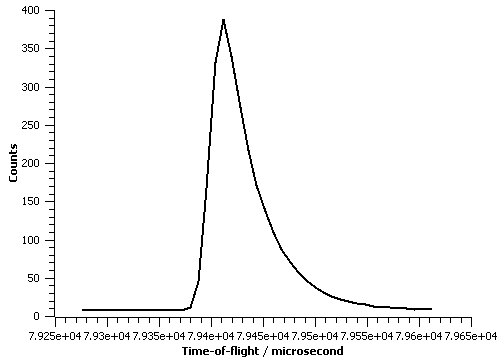$$\renewcommand\AA{\unicode{x212B}}$$

# BackToBackExponential¶

## Description¶

A back-to-back exponential peakshape function is defined as:

$I\frac{AB}{2(A+B)}\left[ \exp \left( \frac{A[AS^2+2(x-X0)]}{2}\right) \mbox{erfc}\left( \frac{AS^2+(x-X0)}{S\sqrt{2}} \right) + \exp \left( \frac{B[BS^2-2(x-X0)]}{2} \right) \mbox{erfc} \left( \frac{[BS^2-(x-X0)]}{S\sqrt{2}} \right) \right].$

This peakshape function represent the convolution of back-to-back exponentials and a Gaussian function and is designed to be used for the data analysis of time-of-flight (TOF) neutron powder diffraction data, see Ref. 1.

The parameters $$A$$ and $$B$$ represent the absolute value of the exponential rise and decay constants (modelling the neutron pulse coming from the moderator) and $$S$$ represent the standard deviation of the Gaussian. The parameter $$X0$$ is the location of the peak; more specifically it represent the point where the exponentially modelled neutron pulse goes from being exponentially rising to exponentially decaying. In general $$X0$$ does not conicide with the maximum of the peak (it is typically at slightly lower TOF than the maximum, by an amount that depends on $$A$$, $$B$$ and $$S$$). $$I$$ is the integrated intensity.

For information about how to convert Fullprof back-to-back exponential parameters into those used for this function see CreateBackToBackParameters. For information about how to create parameters from a GSAS parameter file see CreateBackToBackParametersGSAS.

References

1. R.B. Von Dreele, J.D. Jorgensen & C.G. Windsor, J. Appl. Cryst., 15, 581-589, 1982

The figure below illustrate this peakshape function fitted to a TOF peak:## Properties (fitting parameters)¶

Name Default Description
I 0.0 integrated intensity of the peak
A 1.0 exponential constant of rising part of neutron pulse
B 0.05 exponential constant of decaying part of neutron pulse
X0 0.0 peak position
S 1.0 standard deviation of gaussian part of peakshape function

Note

the initial default guesses for in particular A and B are only based on fitting a couple of peaks in a dataset collected on the ISIS’s HRPD instrument.

Categories: FitFunctions | Peak